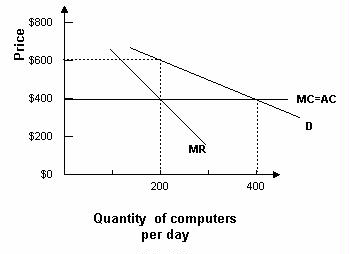### CFA Practice Question

There are 539 practice questions for this study session.

### CFA Practice Question

Refer to the graph below. Assuming that the monopoly maximizes profit, it will earn profits of ______.A. \$8,000 per day
B. \$40,000 per day
C. \$160,000 per day
Explanation: Total profits equal per unit profits (\$200) multiplied by output (200), or \$40,000.

User Comment
jgraham6 can someone help me w/ this??
StanleyMo hello jgraham, the profit is equal price set - ATC area. where you get 200 x (600-400).
Candy220 A monopoly's economic profit is maximized when MR = MC (when the 2 curves intersect). So the profit maximizing output = 200 and the price = \$600. The economic profit is the box where price is greater than AC. So, your profit per output = \$600 - \$400 = \$200 and your profit maximizing ouput = 200, so the economic profit = \$200 * 200 = \$40,000. You can refer to your Economic book (volume 2) pgs 185 - 188 for a better explanation